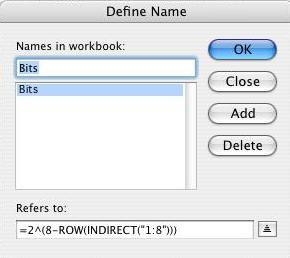#### Mac XL

This site will look better if you upgrade to a browser that supports web standards.

## Bitwise Logical Operations

These functions are shamelessly lifted from a series of posts by Harlan Grove.

XL's comparison operators use the values of their arguments as boolean inputs. For instance =AND(A1, B1) will evaluate each argument for logical TRUE (A1<>0) or FALSE (A1=0), then perform the AND, returning either TRUE or FALSE. For instance, if A1 is 13 and B1 is 7, =AND(A1,B1) will evaluate that as =AND(TRUE,TRUE) and return TRUE.

In some circumstances, notably programming, being able to do a binary bit-wise operation is desired. For instance, if A1 is 13 and B1 is 7 a bitwise AND would look at each bit and return the logical AND of those bits (or decimal 5):

```A1:     00001101
B1:     00000111
--------
result: 00000101```

### Defining number of bits

These function operate on a fixed number of bits. Depending on the number of bits desired, use Insert/Name/Define to define the constant Bits something like:

```8-bits        =2^(8-ROW(INDIRECT("1:8")))
16-bits       =2^(16-ROW(INDIRECT("1:16")))
32-bits       =2^(32-ROW(INDIRECT("1:32")))```### The logical functions

For integers x and y (or references to cells containing integers):

#### NOT x:

Result bit is 1 if x-bit is 0.

`=SUMPRODUCT(1-MOD(INT(x/Bits),2),Bits)`
```x:      00001101   (decimal 13)
--------
result: 11110010   (decimal 242)```

#### x AND y:

Result bit is 1 if x-bit and y-bit are both 1.

`=SUMPRODUCT(MOD(INT(x/Bits),2)*MOD(INT(y/Bits),2),Bits)`
```x:      00001101    (decimal 13)
y:      00000111    (decimal 7)
--------
result: 00000101    (decimal 5)```

#### x OR y:

Result bit is 1 if x-bit is 1 or y-bit is 1, or both are 1.

`=SUMPRODUCT(SIGN(MOD(INT(x/Bits),2)+MOD(INT(y/Bits),2)),Bits)`
```x:      00001101    (decimal 13)
y:      00000111    (decimal 7)
--------
result: 00001111    (decimal 15)```

#### x XOR y:

Result bit is 1 if x-bit is 1 or y-bit is 1, but not if both are 1.

`=SUMPRODUCT(MOD(MOD(INT(x/Bits),2)+MOD(INT(y/Bits),2),2),Bits)`
```x:      00001101    (decimal 13)
y:      00000111    (decimal 7)
--------
result: 00001010    (decimal 10)```

#### x IMP y:

Result bit is 1 if y-bit is 1 or if both x-bit and y-bit are 0.

`=SUMPRODUCT(1-(MOD(INT(x/Bits),2)*(1-MOD(INT(y/Bits),2))),Bits)`
```x:      00001101    (decimal 13)
y:      00000111    (decimal 7)
--------
result: 11110111    (decimal 247)```

#### x EQ y:

Result bit is 1 if x-bit and y-bit are equal.

`=SUMPRODUCT(1-MOD(MOD(INT(x/Bits),2)+MOD(INT(y/Bits),2),2),Bits)`
```x:      00001101    (decimal 13)
y:      00000111    (decimal 7)
--------
result: 11110101    (decimal 245)```